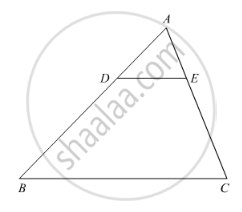Share

# In the Following Figure, De || Bc Such that Ae = (1/4) Ac. If Ab = 6 Cm, Find Ad. - CBSE Class 10 - Mathematics

ConceptCriteria for Similarity of Triangles

#### Question

In the following Figure, DE || BC such that AE = (1/4) AC. If AB = 6 cm, find AD.#### Solution

We have, DE || BC, AB = 6 cm and AE = 1/4 AC

∠A = ∠A                          [Common]

Then, ΔADE ~ ΔABC         [By AA similarity]

rArr"AD"/"AB"="AE"/"AC"             [Corresponding parts of similar Δ are proportional]

rArr"AD"/6=(1/4AC)/"AC"                [∵ AE =1/4 AC given]

"AD"/6=1/4

rArr"AD"=6/4=1.5 cm

Is there an error in this question or solution?

#### Video TutorialsVIEW ALL 

Solution In the Following Figure, De || Bc Such that Ae = (1/4) Ac. If Ab = 6 Cm, Find Ad. Concept: Criteria for Similarity of Triangles.
S